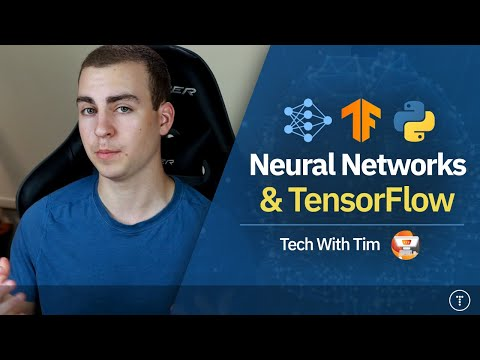In this 2+ hour crash course, we will dive into neural networks and the TensorFlow Python library

There are text based tutorials for each part of this video. You can find them all here:

Python Neural Networks

Tensorflow install: pip install -q tensorflow==2.0.0-alpha0

Tensorflow Guides Followed:
https://www.tensorflow.org/tutorials/keras/classification
https://www.tensorflow.org/tutorials/keras/text_classification

Timestamps: before intro
00:00:00 – Introduction
00:00:27 – How a Neural Network Works
00:37:44 – Building Our First Model
00:55:05 – Making Predictions
01:00:09 – Text Classification with Movie Reviews
01:26:40 – Embedding Layer Explanation
01:35:55 – Global Average Pooling Layer
01:40:23 – Training the Text Classification Model